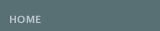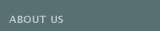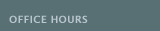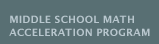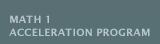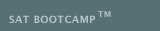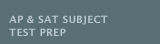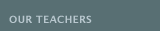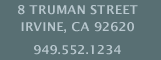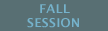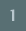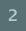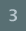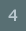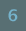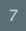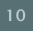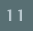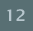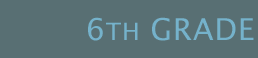The STRUCTURED WRITING FOR THE 6th GRADE is a small group class that provides instruction aligned with the California's Common Core State Standards in the following areas: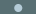Active Reading (Reading Comprehension): Students will read and understand 4-5 novels, cite textural evidence to support analysis and inferences drawn from the text, determine a theme and how it is conveyed through particular details, provide a summary distinct from personal opinions, analyze how a particular sentence, chapter or scene fits into the overall development of the theme, setting, or plot and explain the effects of common literary devices (e.g., symbolism, imagery, metaphor).Fundamentals of Effective Writing: Students will be writing clear, coherent, and focused essays that introduce a thesis statement, organize ideas using strategies such as definition, classification, comparison/contrast, and cause/effect, develop a topic with relevant facts, quotations, and definitions, use appropriate transitions to clarify the relationships among ideas and concepts, establish and maintain a formal style, provide a concluding paragraph. Students will be practicing the three writing genres (expository, persuasive arguments, and descriptive narratives) to include at least 500�800 words in each composition.Vocabulary Acquisition: Students will integrate knowledge of individual words to enhance their writing, identify and interpret figurative language and words with multiple meanings, and understand and explain "shades of meaning" in related words.Grammar: For the grammar module of the class, students will learn to identify and properly use all pronouns (e.g., subjective, objective, possessive case) and present perfect, past perfect, and future perfect verb tenses, ensure that verbs agree with compound subjects, use simple, compound, and compound-complex sentences, use effective coordination and subordination of ideas to express complete thoughts and the appropriate use of colons and semicolons to connect independent clauses. Some book purchases will be necessary. Students can expect homework and weekly quizzes. Grades will be given.
 E06B STRUCTURED WRITING (6th Gr) August 19�January 24 (23 weeks)* *October 14 (Columbus Day Observed) *November 25�30 (Thanksgiving Recess)
 Wednesdays 4:00�6:00pm HUGHES
 The BARUN ACADEMIC CENTER's APPLIED MATH for the 6th GRADE provides an excellent foundation aligned with California's COMMON CORE State Standards for the aspiring math student. The curriculum provides students an opportunity to achieve the following broad instructional goals:Ratios and Proportional Relationships: Use ratio and rate reasoning to solve real-world and mathematical problems (by reasoning about tables of equivalent ratios, tape diagrams, double number line diagrams, or equations).The Number System: Fluently add, subtract, and divide multi-digit numbers and decimals using the standard algorithm for each operation. Understand ordering and absolute value of rational numbers. Solve real-world and mathematical problems by graphing points in all four quadrants of the coordinate plane.Expressions and Equations: Evaluate expressions at specific values of their variables. Reason about and solve one-variable equations and inequalities. Represent and analyze quantitative relationships between dependent and independent variables.Geometry: Solve real-world and mathematical problems involving area, surface area, and volume.Statistics and Probability: Develop understanding of statistical variability. Display numberical data in plots on a number line, including dot plots, histograms, and box plots. This group class is for the 5th grade APAAS or 6th grade student. Students can expect homework, quizzes, a mid-term and a final. Grades will be given.
 M60A APPLIED MATH (6th Gr) August 19�January 24 (23 weeks)* *October 14 (Columbus Day Observed) *November 25�30 (Thanksgiving Recess)
 The MIDDLE SCHOOL MATH ACCELERATION PROGRAM (MSMAP) at the BARUN ACADEMIC CENTER is designed to prepare the 6th grade student for the appropriate advanced math class.
 APPLIED MATH FOR THE 6TH GRADE (MSMAP) is designed for the 6th grade student for Common Core ENHANCED Math 7/8 placement in the 7th grade. This group class is aligned with the California's COMMON CORE State Standards:Ratios and Proportional Relationships: Use ratio and rate reasoning to solve real-world and mathematical problems (by reasoning about tables of equivalent ratios, tape diagrams, double number line diagrams, or equations).The Number System: Fluently add, subtract, and divide multi-digit numbers and decimals using the standard algorithm for each operation. Understand ordering and absolute value of rational numbers. Solve real-world and mathematical problems by graphing points in all four quadrants of the coordinate plane.Expressions and Equations: Evaluate expressions at specific values of their variables. Reason about and solve one-variable equations and inequalities. Represent and analyze quantitative relationships between dependent and independent variables.Geometry: Solve real-world and mathematical problems involving area, surface area, and volume.Statistics and Probability: Develop understanding of statistical variability. Display numerical data in plots on a number line, including dot plots, histograms, and box plots.
 Students will be required to attend twice a week (one lesson to emphasize problem solving and word problems and the other to reinforce accuracy). Students are expected to complete additional exercise problem sets for homework. CAASPP test preparation will be integrated in the weekly lectures. Students can expect homework, quizzes, a mid-term and a final. Grades will be given.
 All sixth graders will be taking the Middle SchoolMath Acceleration Test in the Spring.
 M60A Applied Math 6 (ENHANCED MATH 7/8 MSMAP)
 August 19�June 5 (42 weeks)* *October 14 (Columbus Day Observed) *November 25�30 (Thanksgiving Recess) *March 30�April 5 (Spring Recess)
 December 2�April 24 (21 weeks)* *October 14 (Columbus Day Observed) *March 30�April 5 (Spring Recess)
 The MIDDLE SCHOOL MATH ACCELERATION PROGRAM (MSMAP) at the BARUN ACADEMIC CENTER is designed to prepare the 6th grade student for the appropriate advanced math class.
 APPLIED MATH FOR THE 6TH GRADE (MSMAP) is designed for the select 6th grade student for MATH 1 INTEGRATED placement in the 7th grade. This group class is aligned with the California's COMMON CORE State Standards:Ratios and Proportional Relationships: Use proportional relationships to solve multistep ratio and percent problems.Expressions and Equations: Use variables to represent quantities in a real-world or mathematical problem, and construct equations and inequalities to solve problems by reasoning about the quantities.Geometry: Use facts about supplementary, complementary, vertical, and adjacent angles in a multi-step problem to write and solve equations for an unknown angle in a figure. Solve real-world problems involving area, volume and surface area of 2 and 3 dimensional objects composed of triangles, quadrilaterals, polygons, cubes, and right prisms.Statistics and Probability: Investigate chance processes and develop, use, and evaluate probability models. Develop a probability model and use it to find probabilities of events.
 Students will be required to attend twice a week (one lesson to emphasize problem solving and word problems and the other to reinforce accuracy). Students are expected to complete additional exercise problem sets for homework. CAASPP test preparation will be integrated in the weekly lectures. Students can expect homework, quizzes, a mid-term and a final. Grades will be given.
 All sixth graders must first pass the Middle School Math Acceleration Testto take the Math 1 Integrated Acceleration Test in the Spring.
 M00A Math 7 (Math 1 Int Placement-MSMAP)
 August 19�June 5 (42 weeks)* *October 14 (Columbus Day Observed) *November 25�30 (Thanksgiving Recess) *March 30�April 5 (Spring Recess)
 Thursdays 4:00-6:00pm KOZICK
 December 2�April 24 (21 weeks)* *October 14 (Columbus Day Observed) *March 30�April 5 (Spring Recess)
 Mondays or Wednesdays 4:00-6:00pm KOZICK